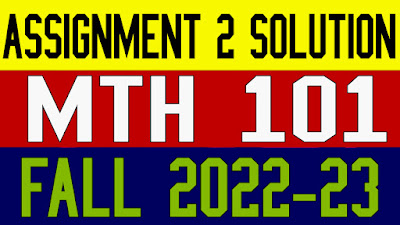### Latest Post

6/recent/ticker-posts

# MTH101 Assignment 2 Solution Fall 2022

MTH101 Assignment 2 Solution Fall 2022: Today we are sharing with you mth101 assignment 2 solution fall 2022 before creating your assignment & gdb file please read all the instructions carefully and follow the rules.Mth101 Assignment 2 Solution Fall 2022

Algebra is a branch of Mathematics that uses mathematical statements to describe relationships between things that vary over time. These variables include things like the relationship between supply of an object and its price. When we use a mathematical statement to describe a relationship, we often use letters to represent the quantity that varies, since it is not a fixed amount. These letters and symbols are referred to as variables.

In algebra, letters are sometimes used in place of numbers. The mathematical statements that describe relationships are expressed using algebraic terms, expressions, or equations (mathematical statements containing letters or symbols to represent numbers). Before we use algebra to find information about these kinds of relationships, it is important to first introduce some basic terminology.

Important Instructions:

·        To solve the assignment you should have good command over 1 - 10 lectures.

·        Don't use colorful background in your solution file.

·        use math type or Equation Editor Etc. for mathematical symbols.